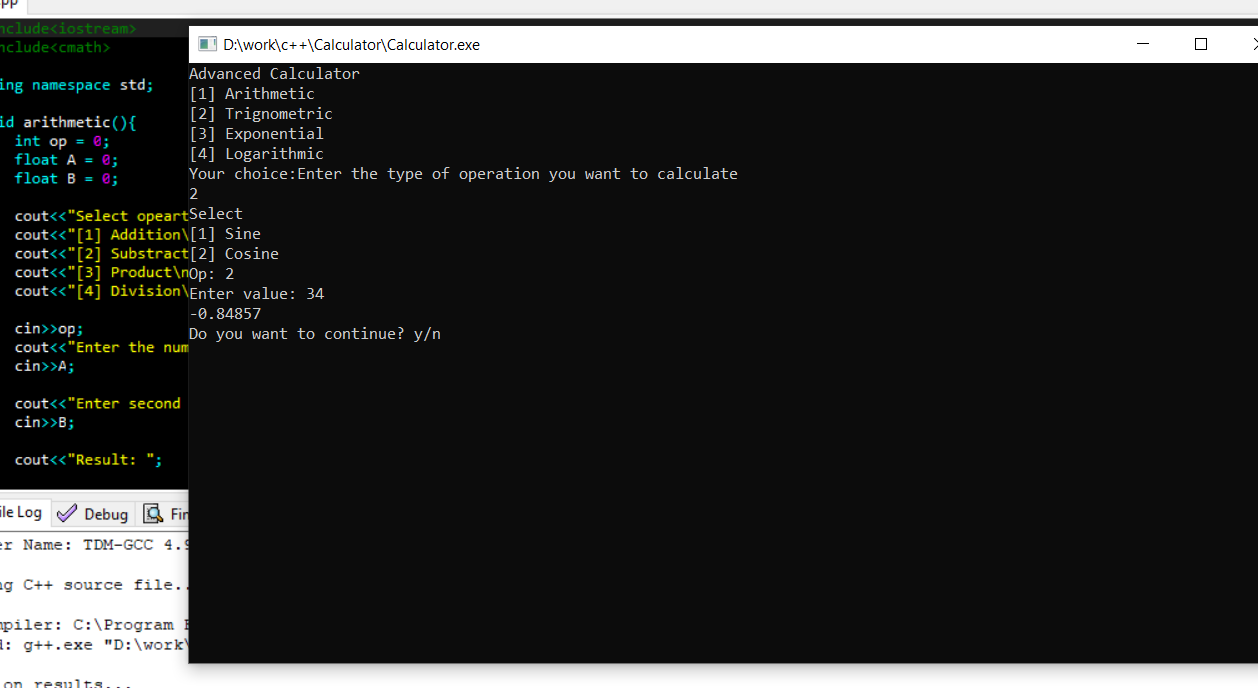# Advance Calculator In C++ With Source Code

Project: Advance Calculator In C++ With Source Code

The Advance Calculator is based on the concept of different mathematical calculations. This whole system is in the C++ language. Here, the user can perform various calculations like arithmetic, trigonometric, exponential, and logarithmic. Also, it is a very effective and simple understanding of beginners. Thus, this is a simple mini project in C++, built as a console application without using any graphics features.

This Advance Calculator project is designed in ‘C++’ language. This system is based on a concept to generate the total sum, subtractions, divisions, multiplications, trigonometric values, and many more. Also, this System makes easy to calculate different numbers. Here, the user has to input the numbers and the system will display output. Also, you can see the basic features of the scientific calculator in this project. Moreover, this mini project is easy to operate and understand by the users.

Features:

•  Arithmetic
•  Trigonometric
•  Exponential
•  Logarithmic

#### How To Run the Project?

In order to run the project, you must have installed Dev C++ or Code Blocks on your PC. (we recommend these IDE). For the project demo, have a look at the video below:

If stuck or need help customizing this project as per your need, just comment down below and we will do our best to answer your question ASAP.